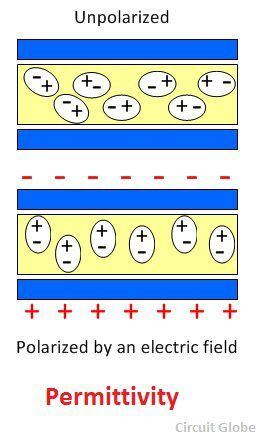# Difference Between Permittivity & Permeability

One of the major difference between the permittivity and the permeability is that the permittivity measures the obstruction generates by the material in the formation of an electric field. Whereas, the permeability measures the ability of the material to allow the magnetic lines of force to pass through it. The other differences between the permittivity and permeability are explained below in the comparison chart.

The permittivity of the material depends on their property of polarisation, and the permeability depends on magnetism.

## Comparison Chart

Basis For ComparisonPermittivity Permeability
Definition The Permittivity measures the resistance offer by the material in the formation of an electric field. The permeability measures the ability of the material to allow the magnetic lines of force to pass through it.
Symbol ε μ
FormulaRatio of displacement field strength to the electric field strength.Ratio of magnetic field density and magnetic field strength.
Physical BasisPolarization Magnetization
Free Space The permittivity of the free space is 8.85 F/m.The permeability of the free space is 1.26 H/m.
Field Electric Field Magnetic Field
Used in Capacitor Inductor and Transformer core

### Definition of Permittivity

The permittivity is the property of the material which measures the opposition generates by the material in the development of an electric field. It is explained as the ratio of the electric displacement to the electric field intensity.The symbol ε represents the permittivity of the material. The SI unit of permittivity is farad per meter. The vacuum has the lowest possible permittivity which is approximately 8.85 X 10-12 Faraday/meter. The permittivity measures the number of charges require in generating one unit of an electric flux in the particular medium.

### Definition of Permeability

Permeability is the property of the material which supports the formation of the magnetic field. The temperature, field strength, field frequency and the humidity affects the permeability of the material. It is represented by μ.The permeability of the material is directly proportional to the conduction of the magnetic lines of force. It is defined as the ratio of the flux density to the field strength of the material. The permeability of the free space is known as the permeability constant. It is represented by μ0 and it is approximately equal to 4Π X 10-7Henry/meter.

## Key Differences between the Permittivity and Permeability

The following are the differences between the permittivity and permeability.

1. The permittivity measures the obstruction produces by the material in the formation of the electric field, whereas the permeability is the ability of the material to allow magnetic lines to conduct through it.
2. The permittivity is represented by ε whereas μ represents the permeability.
3. The SI unit of permittivity is Faraday/meter and that of the permeability is Henry/meter.
4. The permittivity is because of polarization whereas the permeability is because of magnetization.
5. The free space of the permittivity is 8.85 F/m, whereas that of the permeability it is 1.26 H/m.
6. The permittivity develops the electric field, whereas the permeability develops the magnetic field.
7. The high permittivity develops in capacitor whereas the high permeability develops in the inductor and the cores of the transformer.

### Conclusion

The permittivity and permeability both are the concepts of the electromagnetic theory. The permittivity is related to the formation of an electric field, and the permeability is because of the magnetic field.

### 9 thoughts on “Difference Between Permittivity & Permeability”

1. The explanation is very clear. I understood the concepts very easily. I thank you very much from the bottom of my heart.